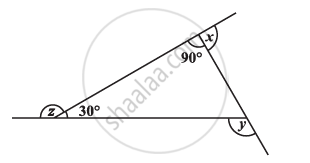Share

# Find X + Y + Z - CBSE Class 8 - Mathematics

ConceptSum of the Measures of the Exterior Angles of a Polygon

#### QuestionFind x + y + z

#### Solution

x + 90° = 180° (Linear pair)

x = 90°

z + 30° = 180° (Linear pair)

z = 150°

y = 90° + 30° (Exterior angle theorem)

y = 120°

x + y + z = 90° + 120° + 150° = 360°

Is there an error in this question or solution?

#### APPEARS IN

NCERT Solution for Mathematics Textbook for Class 8 (2018 to Current)